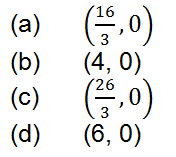# From the point (15, 12) three normals are drawn to the parabola y2 = 4x, then centroid of triangle formed by three co-normal points is9 years ago

(C)

Sol.     Let the equation of any normal be y = – tx + 2t + t3

Since it passes through the points (15, 12)

\         12 = –15t + 2t + t3                 i.e.       t3 – 13t – 12 = 0

\         t = –1, –3/4

\         The points are (1, –2), (9 – 6), (16, 8)        \         Centroid is (26/3,0)